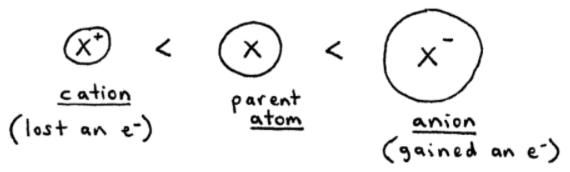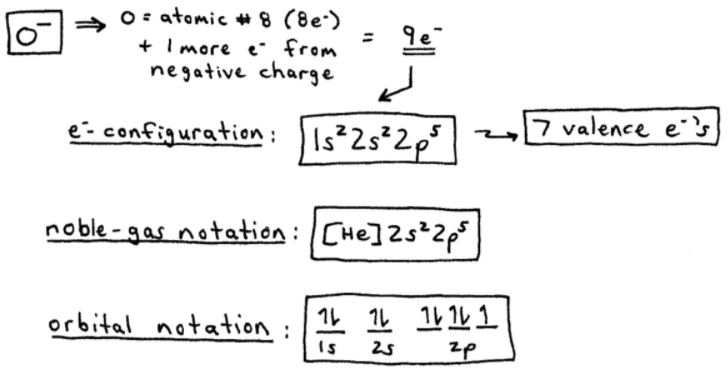# S8E3 - Ion Electron Configurations and Ionic Radius Trends

In this post we'll discuss the relative sizes of ions, and the three ways to write an ion's electron configuration:

- complete electron configuration
- noble-gas notation (shorthand e- configuration)
- orbital notation

----------

## Relative Sizes of Ions

➞  generally, a cation (X+) is smaller than the parent atom (X).

➞  generally, an anion (X-) is larger than the parent atom (X).----------

ex:  Order the ions from smallest to largest ionic radius.

(a)   Li+ ,  Na+ ,  K+ ,  Rb+ ,  Cs+

➞  well... all of these are group 1 cations and size increases down a group, remember?  So we have:

Li+   <   Na+   <   K+   <   Rb+   <   Cs+----------

(b)   Ba2+ ,  Cs+ ,  I- ,  Te2-

➞  in this grouping, it's not so easy as in part (a).

All four ions have 54 e- , so their electron clouds would be the same size.

However, each ion has a different amount of protons (+) in it's nucleus (differing atomic numbers).

The ion with the most protons is best at "pulling" the 54 e- in, toward the nucleus, thus decreasing the size of the electron cloud.

This "pulling" is a direct result of the (+)⇿(-) electrostatic interaction.

Result:

Ba2+   <   Cs+   <   I-   <   Te2-==========

## Ion Electron Configurations

Electron configurations for cations and anions are very similar to the way I taught you previously...

Only now, we have to first add or subtract the proper number of specific electrons.  Here's how:

ex:  Write the electron configuration, noble-gas notation, and orbital notation for O-, Na+, and Fe2+.
_________

➞  to do this, we really only need to know the number of electrons for each ion.

O-   ,   O = atomic number 8, so 8 e-, plus 1 more e- from the negative charge = 9 e-----------
Now, we'll do the same thing for Na+

Na+   ,   Na = atomic number 11, so 11 e-, then 1 less e- from the +1 charge = 10 e-----------
Finally, the last one is the Fe2+ ion...

Fe2+   ,   Fe = atomic number 26, so 26 e-, then 2 less e's from the +2 charge = 24 e-

Beware!  24 electrons is the same number of electrons as the chromium atom, which we know is an exception to the diagonal rule.

So we'll end up with ...4s13d5, as shown below.==========

Whew!  That's a lot of configurations and notations!

Okay, in our next post covering SECTION 8 - Chemical Bonding,

See you on the other side!